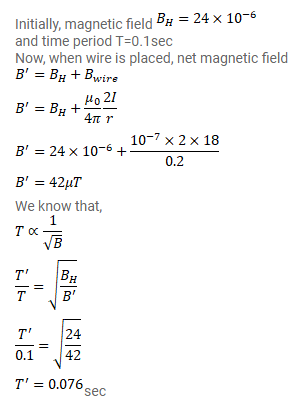# A short magnet oscillates in an oscillation magnetometer with

Question:

A short magnet oscillates in an oscillation magnetometer with a time period of $0.10$ s where the earth's horizontal magnetic field is $24 \mu \mathrm{T}$. A downward current of $18 \mathrm{~A}$ is established in a vertical wire placed $20 \mathrm{~cm}$ east of the magnet. Find the new time period.

Solution: